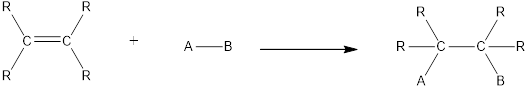Chapter 2.10, Problem 3QQ### Organic And Biological Chemistry

7th Edition
STOKER + 1 other
ISBN: 9781305081079

#### Solutions

Chapter
Section### Organic And Biological Chemistry

7th Edition
STOKER + 1 other
ISBN: 9781305081079
Textbook Problem

# Hydrohalogenation of which of the following alkenes would produce two different halogenated hydrocarbons? a. CH2=CH2 b. CH2=CH—CH3 c. CH3—CH=CH—CH3 d. no correct response

Interpretation Introduction

Interpretation:

Among the given reactions, the one which gives two different halogenated hydrocarbons has to be chosen from the given options.

Concept Introduction:

Chemical reaction in which an atom or a group of atoms are added to each carbon atom of a carbon‑carbon multiple bond in a hydrocarbon or hydrocarbon derivative is known as addition reaction.

In this reaction no atoms or group of atoms are removed. Instead the unsaturated bond is reduced to saturated bond. A general scheme for addition reaction of alkene can be given as shown below,Symmetrical addition reactions is the one in which the same atom or same group of atoms are added across the carbon‑carbon multiple bonds.

Unsymmetrical addition reactions is the one in which the different atom or different group of atoms are added across the carbon‑carbon multiple bonds.

Hydrohalogenation is an unsymmetrical addition reaction. In this reaction a hydrogen atom and a halogen atom are added across the multiple bond. If the alkene considered is a symmetrical one, then only one product is formed. If the alkene considered is an unsymmetrical one, then two products are formed which depends on which side of the double bond the atoms gets attached.

Explanation

Reason for correct option:

The alkene which is an unsymmetrical one produces two products when it undergoes hydrohalogenation reaction. Unsymmetrical alkene is the one where two different alkyl groups are bonded on either side of the double bond. Among the given compounds, it is found that propene is an unsymmetrical alkene. Hence, two products will be formed from this alkene when it undergoes hydrohalogenation.

Therefore, the correct option is option (b)...

### Still sussing out bartleby?

Check out a sample textbook solution.

See a sample solution

#### The Solution to Your Study Problems

Bartleby provides explanations to thousands of textbook problems written by our experts, many with advanced degrees!

Get Started